# AP SSC Board Question Paper for Class 10th Science Paper I 2016 In PDF

## AP 10th Standard Science Paper I Exam Question Papers 2016 with Solutions – Free Download

AP Board Class 10 Science Paper I 2016 Question Paper with solutions is the best resource for students who practise hard to score high in the board exams. This Class 10 exam question paper is useful for the students, as it helps them to perform well in the Science exams. With just a click, students can now download the PDF formats of the solved or the unsolved question paper, as per their preference. These clickable links that are listed below in this article are considered as a very helpful resource to prepare effectively for the exams.

Also, for students who are aspiring to achieve good scores in Science, mastering all the complex concepts related to the subject seems the best possible solution. Students can easily ace the AP Board Class 10 Science Paper I exam, if they have learned the complex formulas and equations of the subject, thoroughly. Students are advised to revise the entire subject before the exam and solve the previous papers of AP Board Class 10 Science Part I. Solving these papers is the best way to get an idea about the exam pattern and help the students become more familiar with the various question types that are normally repeated for board exams. Students will also be able to gauge the difficulty level of the exams from these AP SSC question paper solutions. This will help them to scale their exam preparations.

### AP Board Class 10 Science Part I 2016 Question Paper with Solutions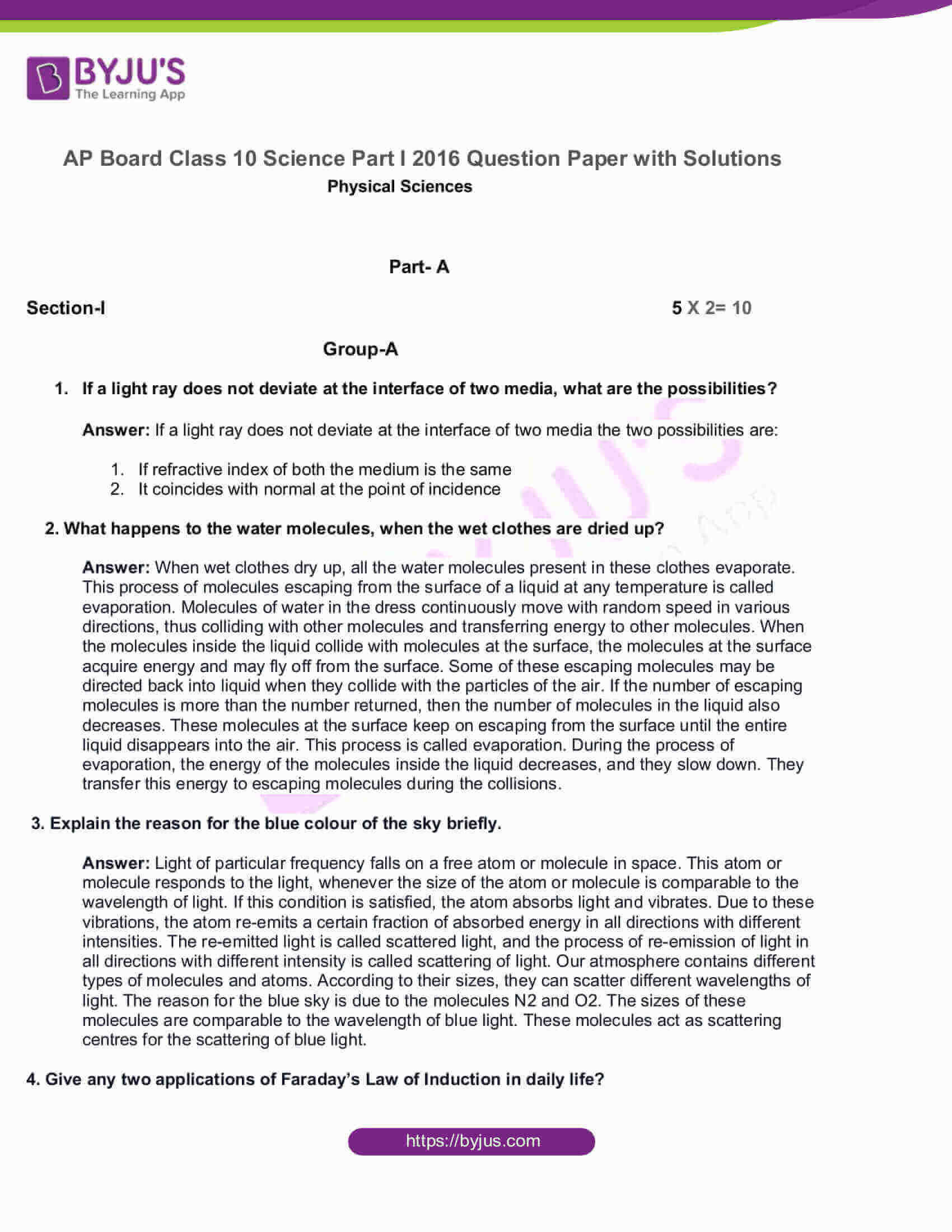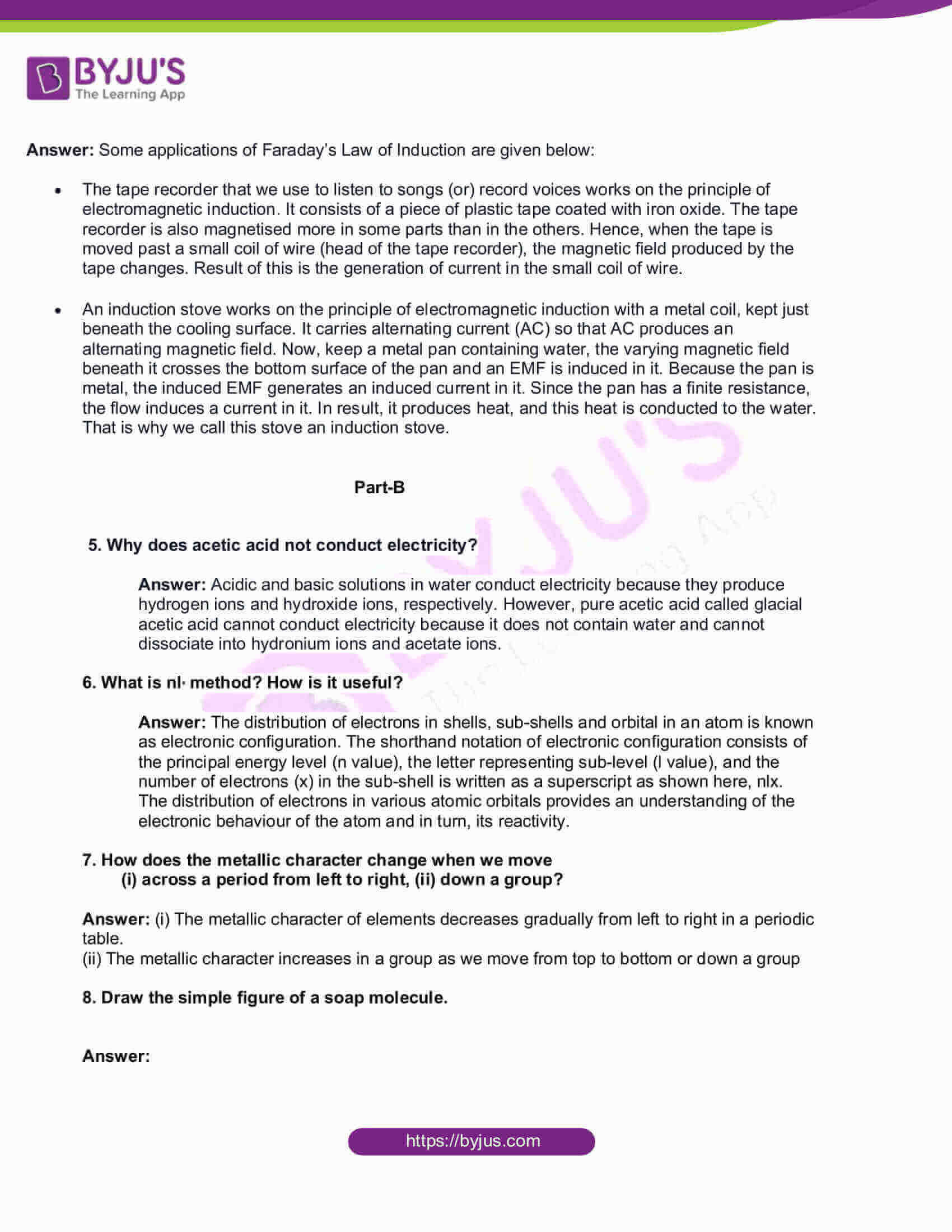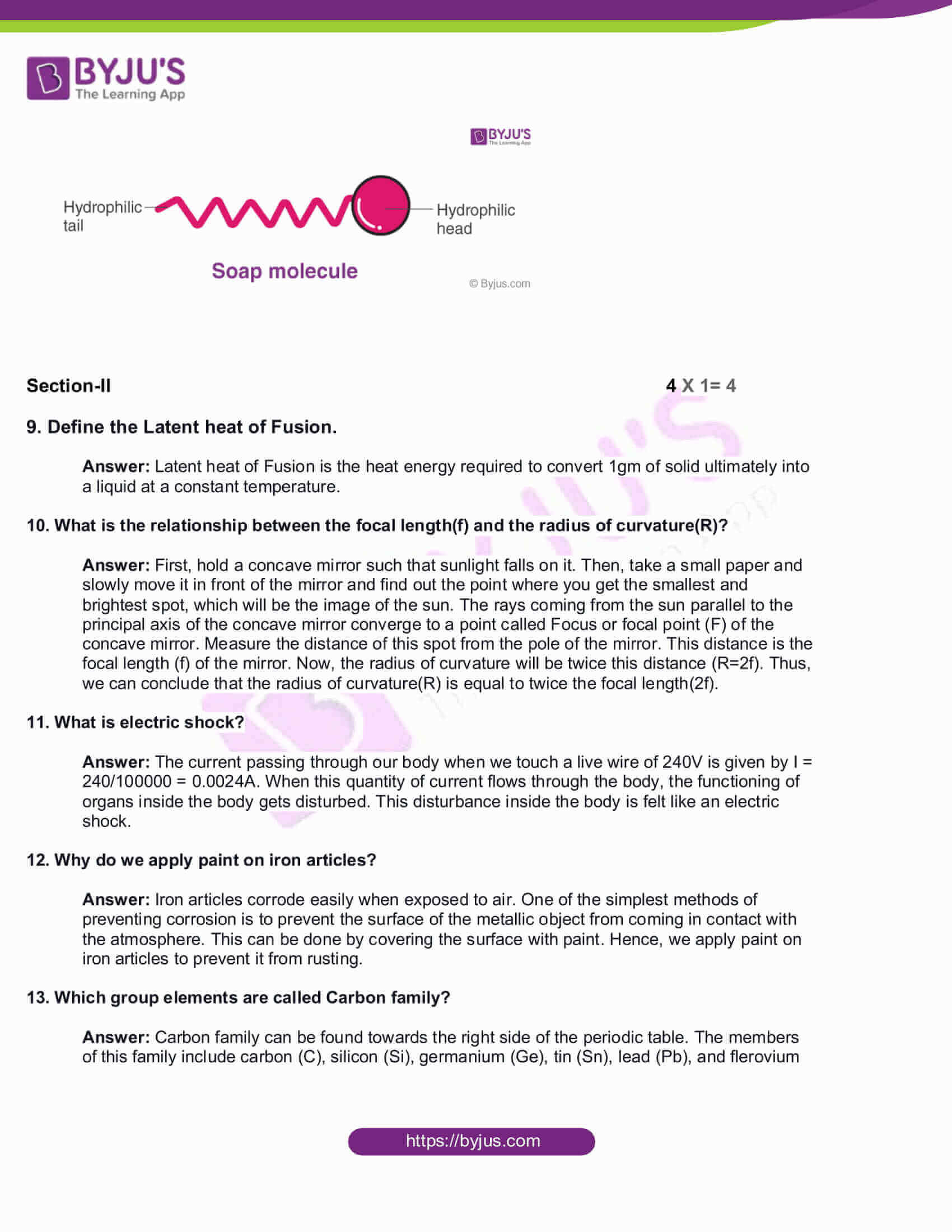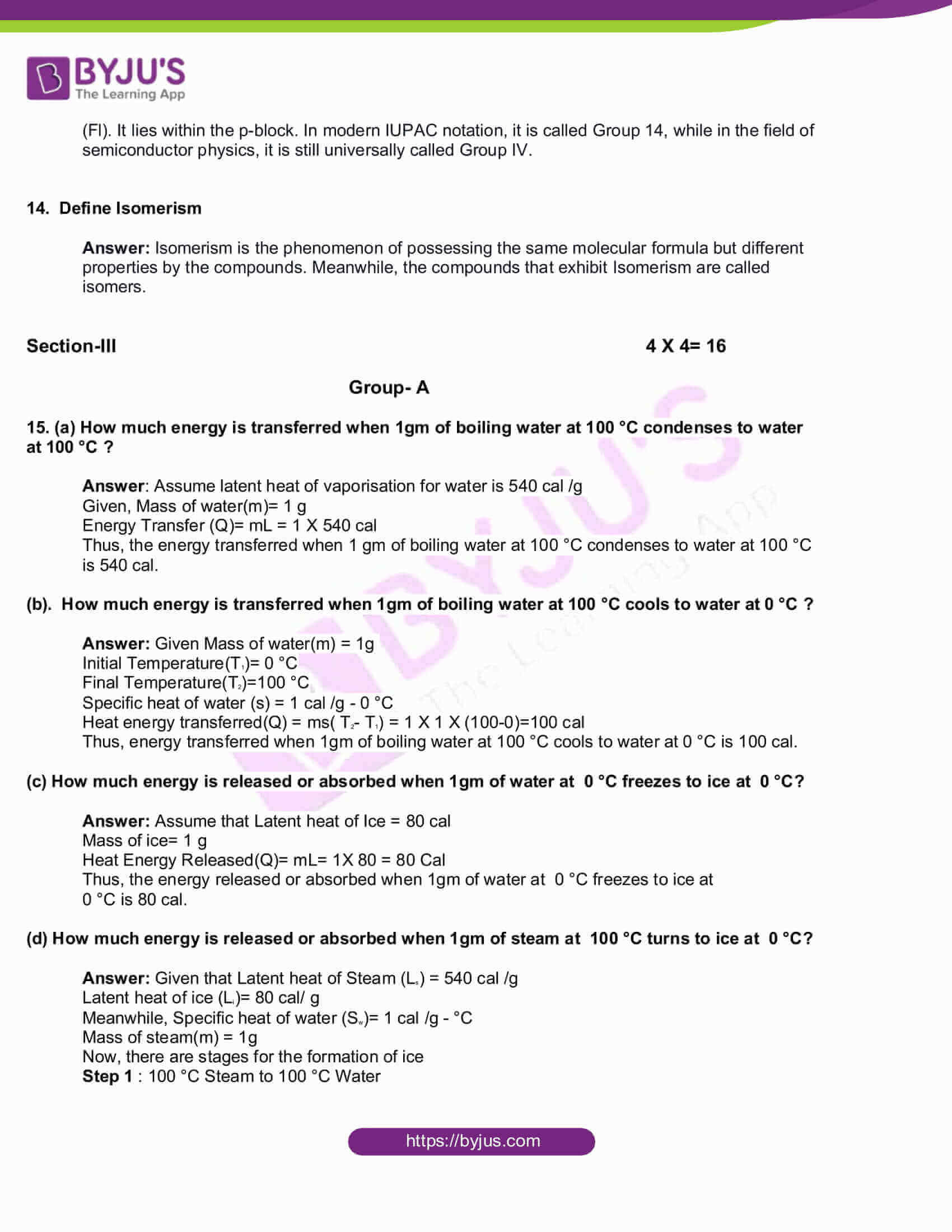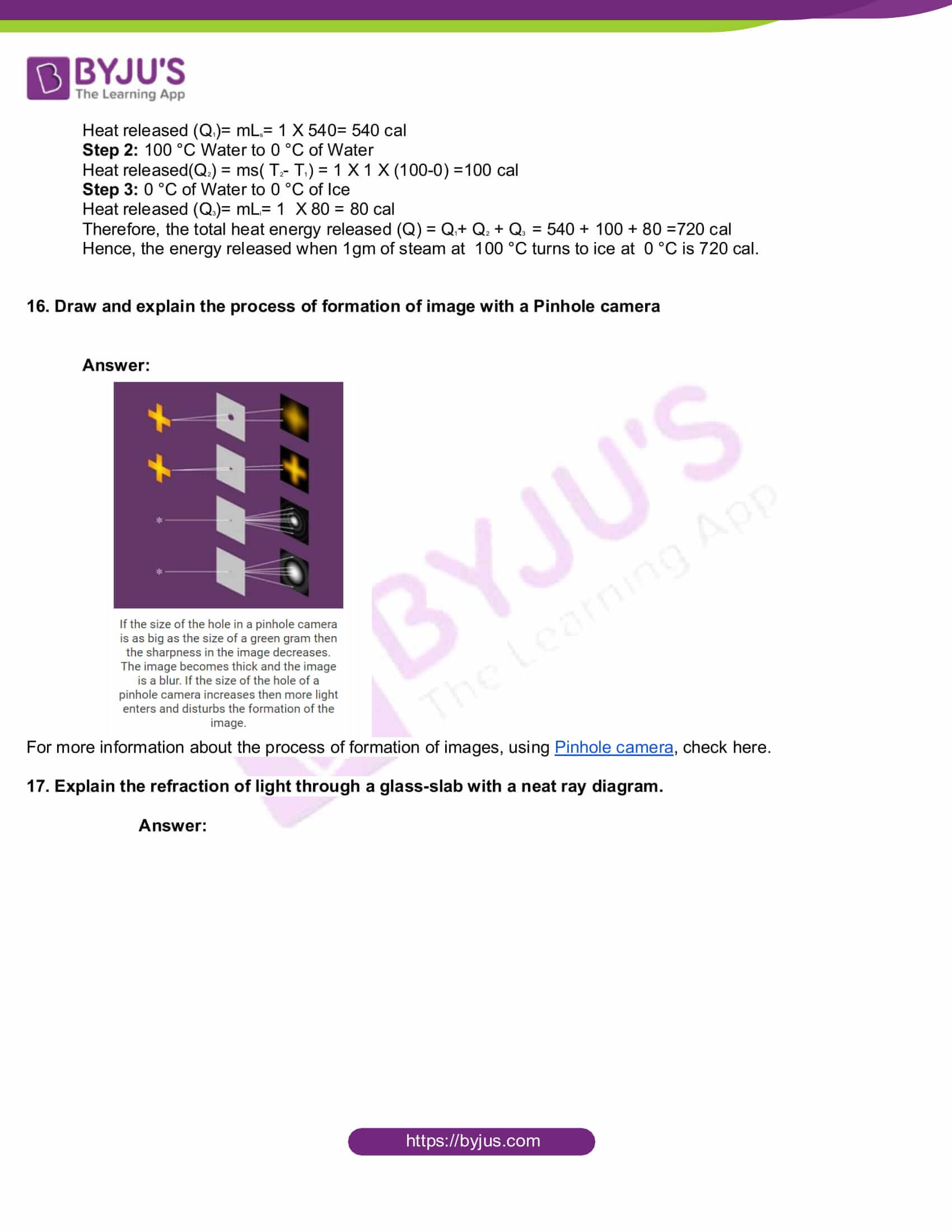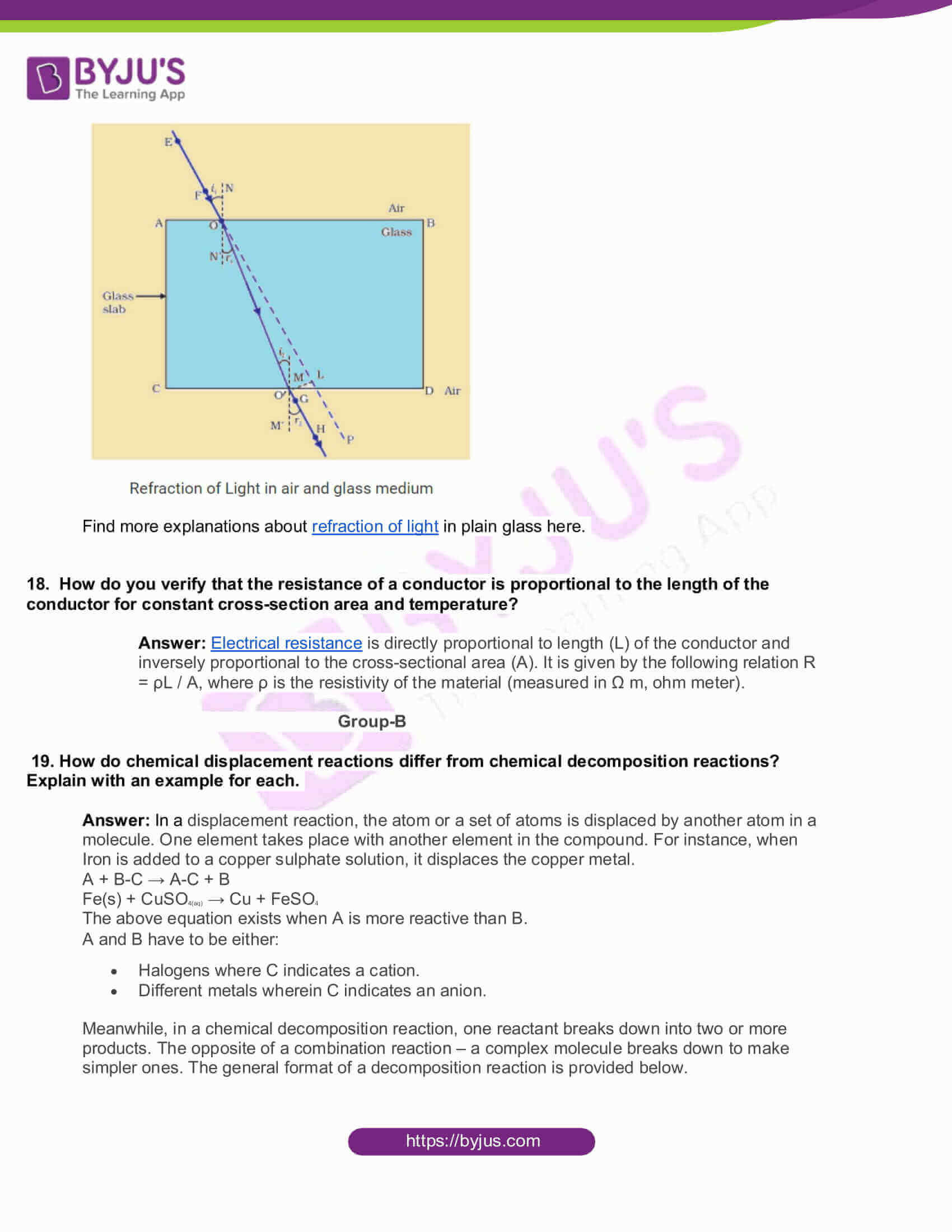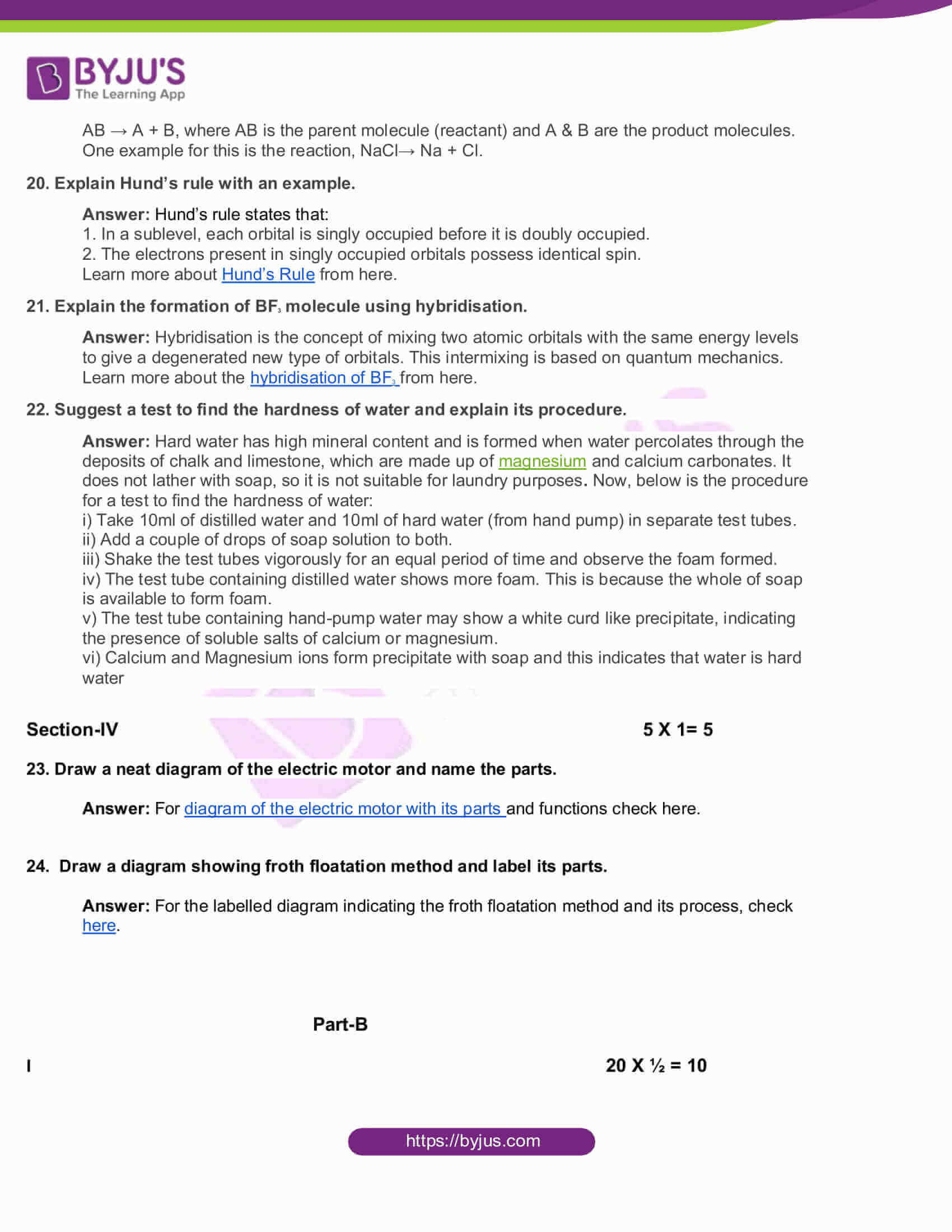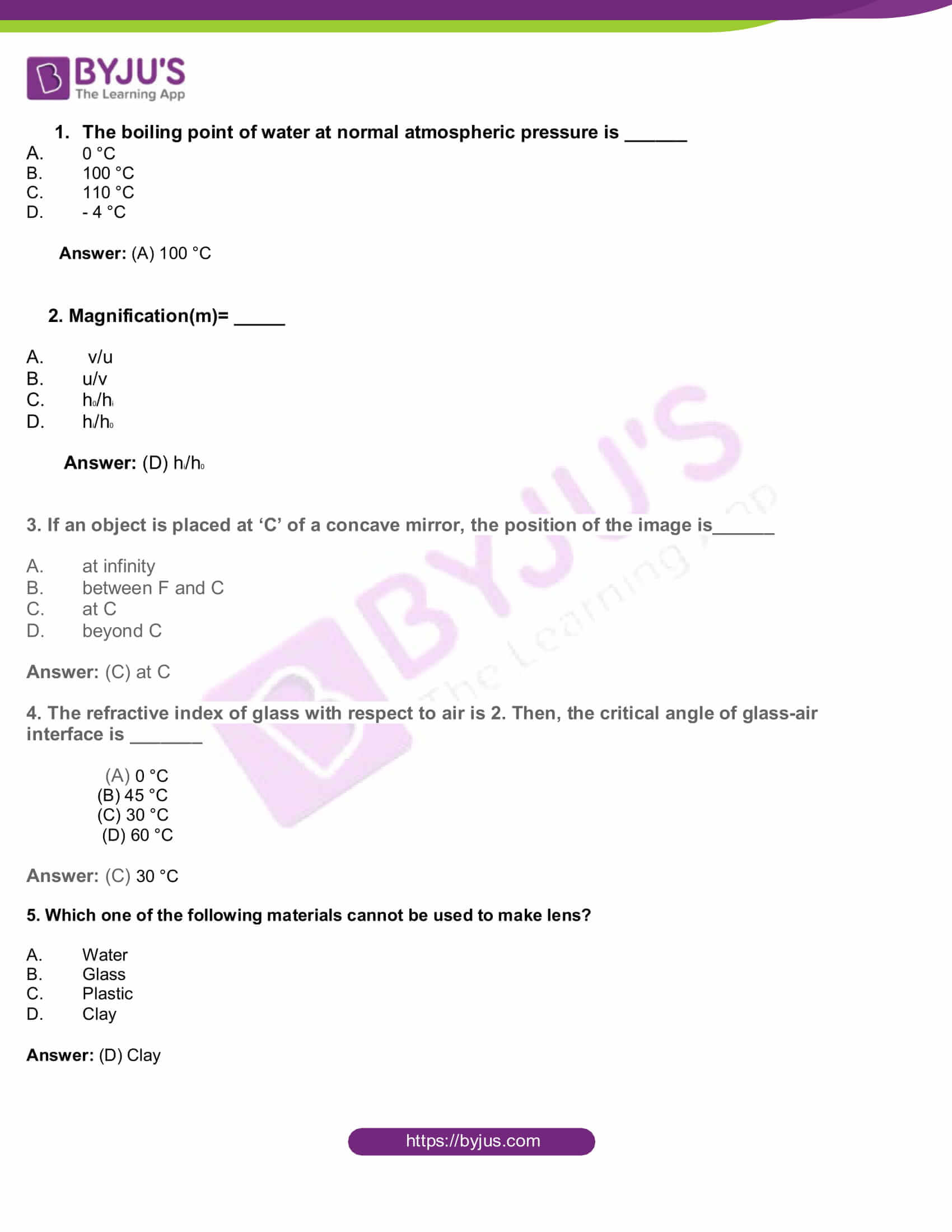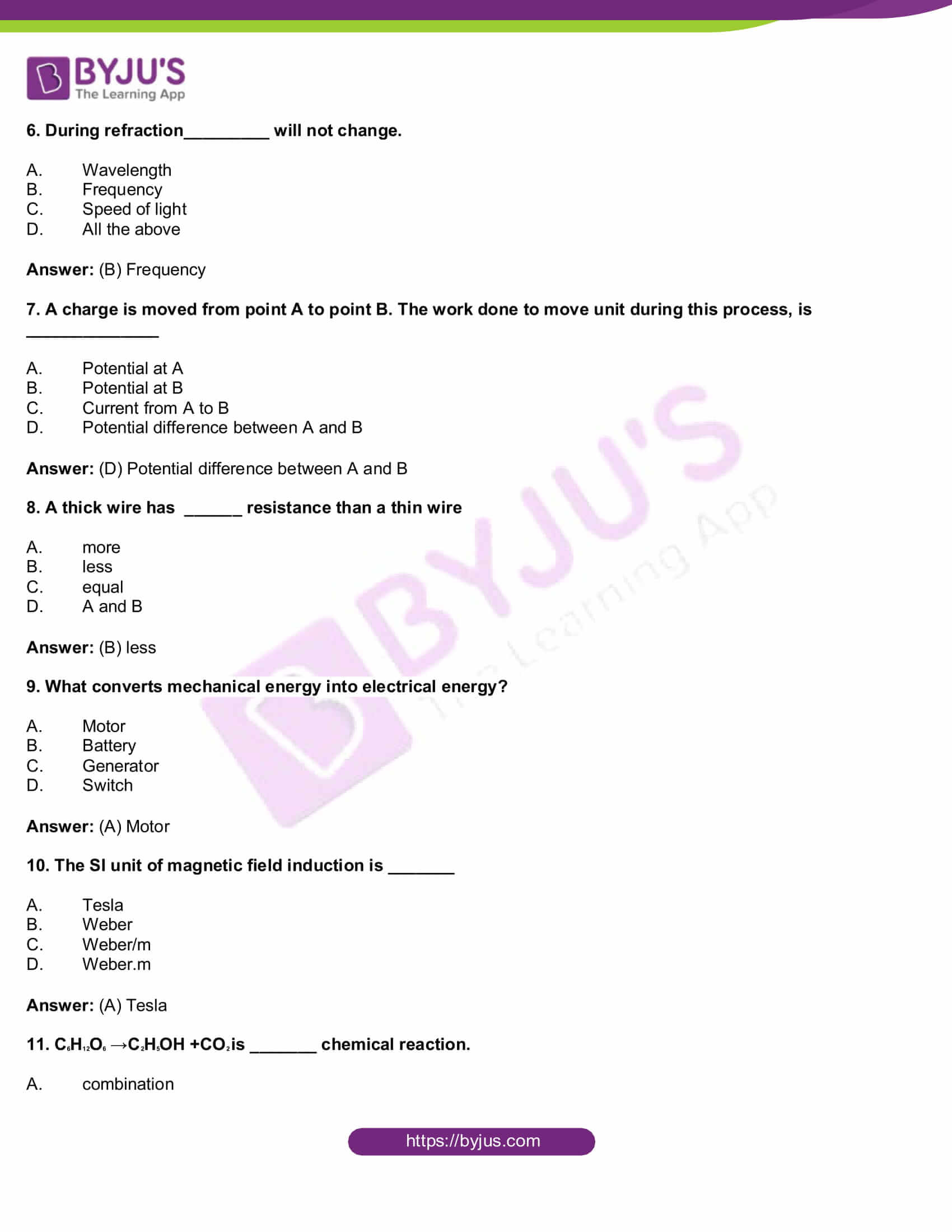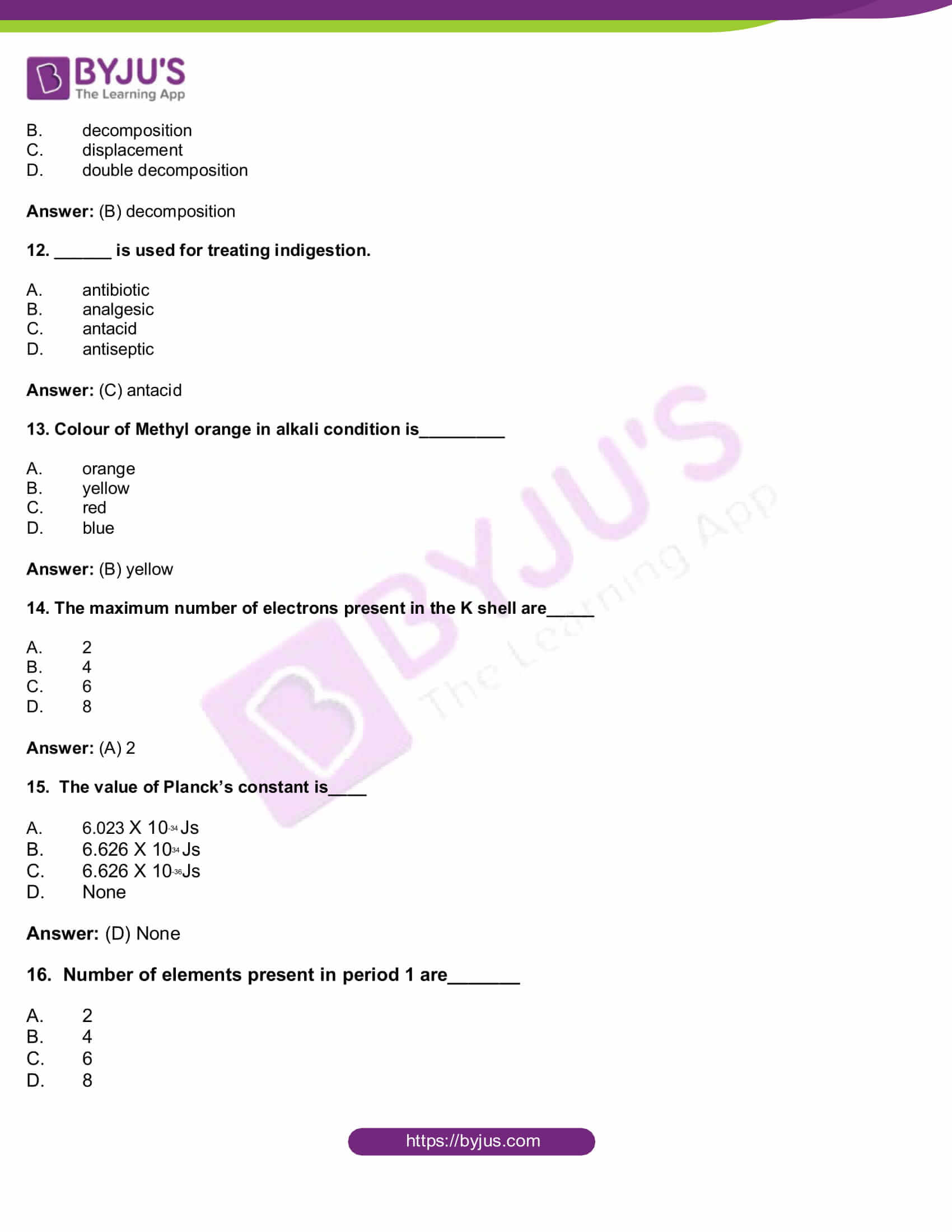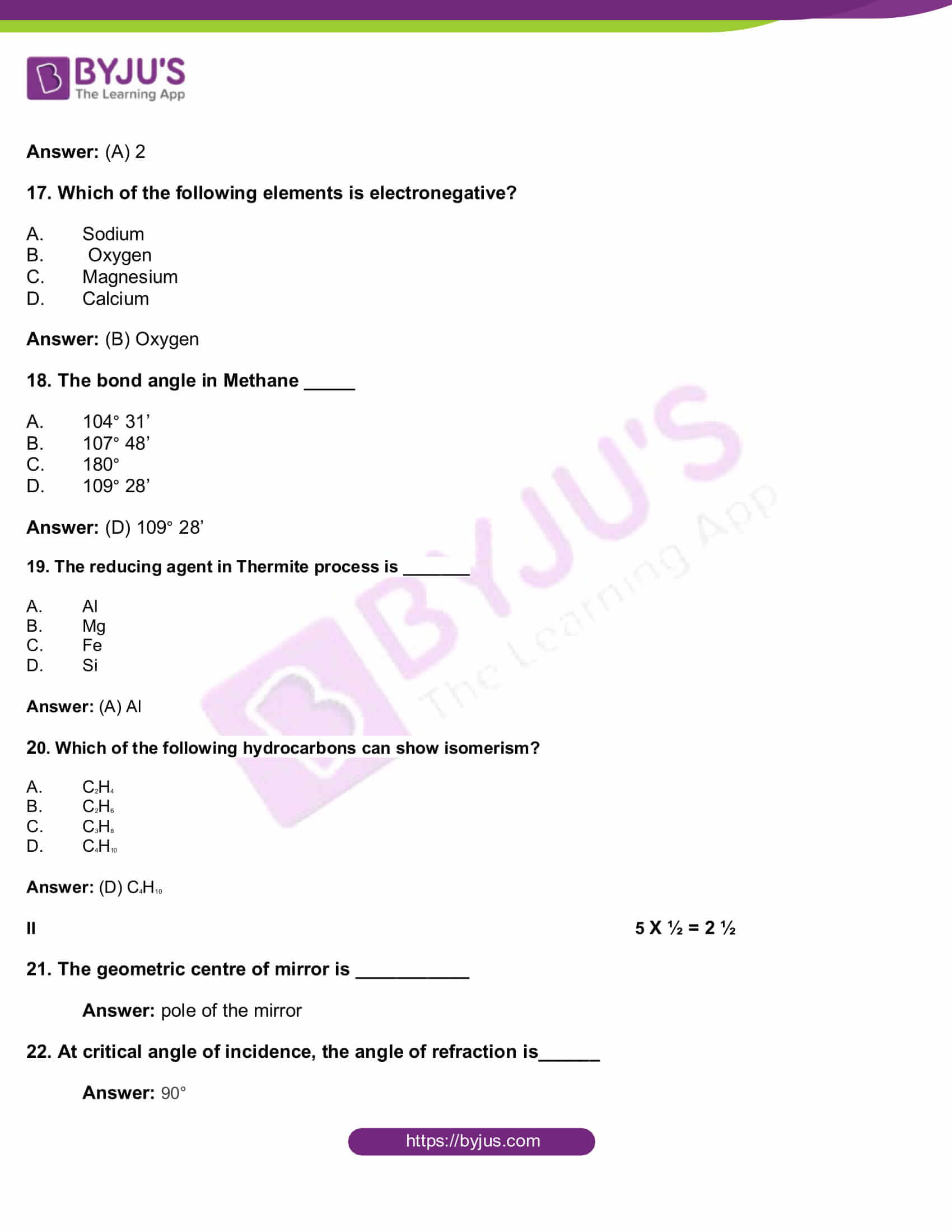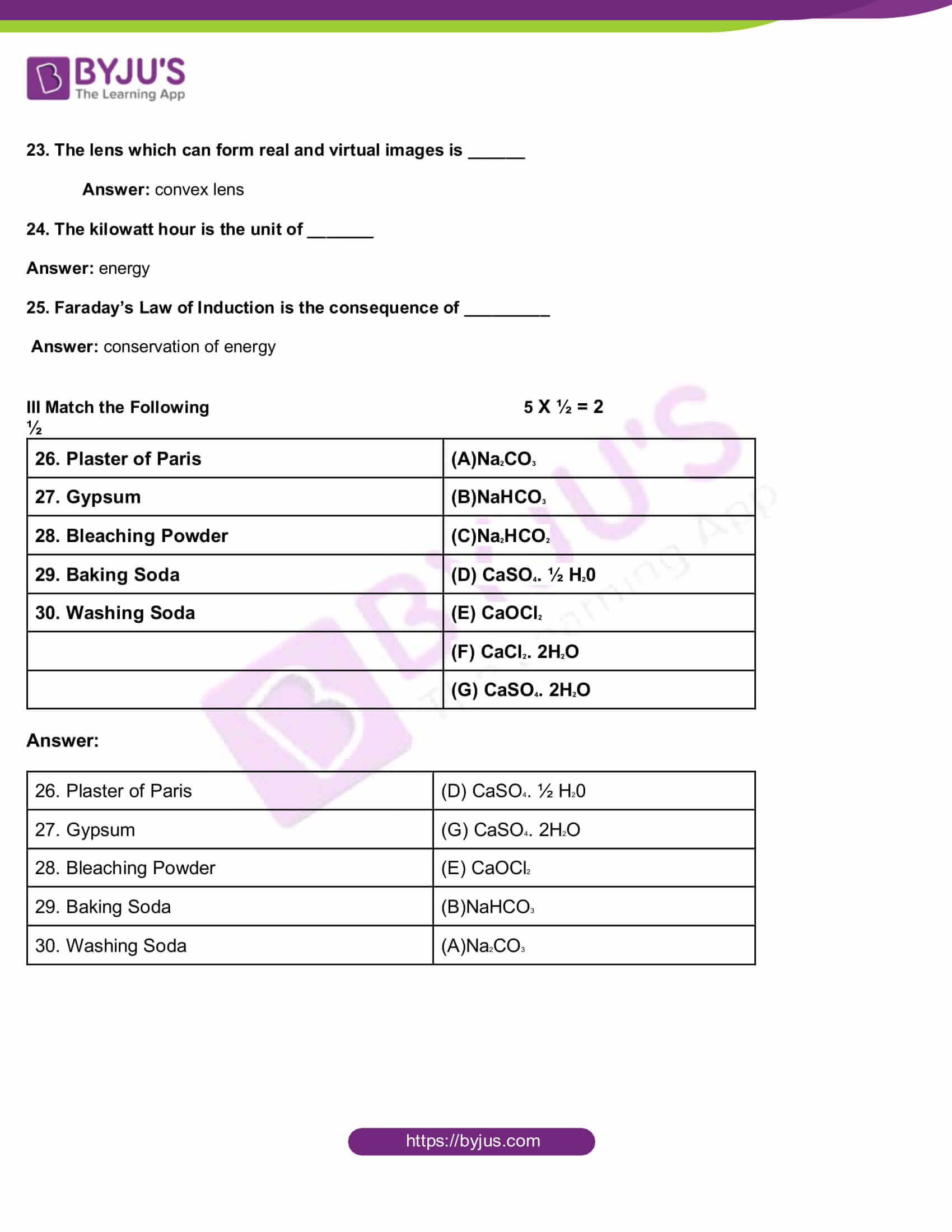Physical Sciences

Part- A

Section-I 5 X 2= 10

Group-A

1. If a light ray does not deviate at the interface of two media, what are the possibilities?

Answer: If a light ray does not deviate at the interface of two media the two possibilities are:

1. If refractive index of both the medium is the same
2. It coincides with normal at the point of incidence

2. What happens to the water molecules, when the wet clothes are dried up?

Answer: When wet clothes dry up, all the water molecules present in these clothes evaporate. This process of molecules escaping from the surface of a liquid at any temperature is called evaporation. Molecules of water in the dress continuously move with random speed in various directions, thus colliding with other molecules and transferring energy to other molecules. When the molecules inside the liquid collide with molecules at the surface, the molecules at the surface acquire energy and may fly off from the surface. Some of these escaping molecules may be directed back into liquid when they collide with the particles of the air. If the number of escaping molecules is more than the number returned, then the number of molecules in the liquid also decreases. These molecules at the surface keep on escaping from the surface until the entire liquid disappears into the air. This process is called evaporation. During the process of evaporation, the energy of the molecules inside the liquid decreases, and they slow down. They transfer this energy to escaping molecules during the collisions.

3. Explain the reason for the blue colour of the sky briefly.

Answer: Light of particular frequency falls on a free atom or molecule in space. This atom or molecule responds to the light, whenever the size of the atom or molecule is comparable to the wavelength of light. If this condition is satisfied, the atom absorbs light and vibrates. Due to these vibrations, the atom re-emits a certain fraction of absorbed energy in all directions with different intensities. The re-emitted light is called scattered light, and the process of re-emission of light in all directions with different intensity is called scattering of light. Our atmosphere contains different types of molecules and atoms. According to their sizes, they can scatter different wavelengths of light. The reason for the blue sky is due to the molecules N2 and O2. The sizes of these molecules are comparable to the wavelength of blue light. These molecules act as scattering centres for the scattering of blue light.

4. Give any two applications of Faraday’s Law of Induction in daily life?

• The tape recorder that we use to listen to songs (or) record voices works on the principle of electromagnetic induction. It consists of a piece of plastic tape coated with iron oxide. The tape recorder is also magnetised more in some parts than in the others. Hence, when the tape is moved past a small coil of wire (head of the tape recorder), the magnetic field produced by the tape changes. Result of this is the generation of current in the small coil of wire.
• An induction stove works on the principle of electromagnetic induction with a metal coil, kept just beneath the cooling surface. It carries alternating current (AC) so that AC produces an alternating magnetic field. Now, keep a metal pan containing water, the varying magnetic field beneath it crosses the bottom surface of the pan and an EMF is induced in it. Because the pan is metal, the induced EMF generates an induced current in it. Since the pan has a finite resistance, the flow induces a current in it. In result, it produces heat, and this heat is conducted to the water. That is why we call this stove an induction stove.

Part-B

5. Why does acetic acid not conduct electricity?

Answer: Acidic and basic solutions in water conduct electricity because they produce hydrogen ions and hydroxide ions, respectively. However, pure acetic acid called glacial acetic acid cannot conduct electricity because it does not contain water and cannot dissociate into hydronium ions and acetate ions.

6. What is nlx method? How is it useful?

Answer: The distribution of electrons in shells, sub-shells and orbital in an atom is known as electronic configuration. The shorthand notation of electronic configuration consists of the principal energy level (n value), the letter representing sub-level (l value), and the number of electrons (x) in the sub-shell is written as a superscript as shown here, nlx. The distribution of electrons in various atomic orbitals provides an understanding of the electronic behaviour of the atom and in turn, its reactivity.

7. How does the metallic character change when we move

(i) across a period from left to right, (ii) down a group?

Answer: (i) The metallic character of elements decreases gradually from left to right in a periodic table.

(ii) The metallic character increases in a group as we move from top to bottom or down a group

8. Draw the simple figure of a soap molecule.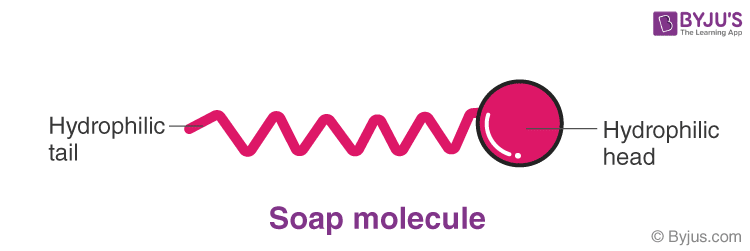Section-II 4 X 1= 4

9. Define the Latent heat of Fusion.

Answer: Latent heat of Fusion is the heat energy required to convert 1gm of solid ultimately into a liquid at a constant temperature.

10. What is the relationship between the focal length(f) and the radius of curvature(R)?

Answer: First, hold a concave mirror such that sunlight falls on it. Then, take a small paper and slowly move it in front of the mirror and find out the point where you get the smallest and brightest spot, which will be the image of the sun. The rays coming from the sun parallel to the principal axis of the concave mirror converge to a point called Focus or focal point (F) of the concave mirror. Measure the distance of this spot from the pole of the mirror. This distance is the focal length (f) of the mirror. Now, the radius of curvature will be twice this distance (R=2f). Thus, we can conclude that the radius of curvature(R) is equal to twice the focal length(2f).

11. What is electric shock?

Answer: The current passing through our body when we touch a live wire of 240V is given by I = 240/100000 = 0.0024A. When this quantity of current flows through the body, the functioning of organs inside the body gets disturbed. This disturbance inside the body is felt like an electric shock.

12. Why do we apply paint on iron articles?

Answer: Iron articles corrode easily when exposed to air. One of the simplest methods of preventing corrosion is to prevent the surface of the metallic object from coming in contact with the atmosphere. This can be done by covering the surface with paint. Hence, we apply paint on iron articles to prevent it from rusting.

13. Which group elements are called Carbon family?

Answer: Carbon family can be found towards the right side of the periodic table. The members of this family include carbon (C), silicon (Si), germanium (Ge), tin (Sn), lead (Pb), and flerovium (Fl). It lies within the p-block. In modern IUPAC notation, it is called Group 14, while in the field of semiconductor physics, it is still universally called Group IV.

14. Define Isomerism

Answer: Isomerism is the phenomenon of possessing the same molecular formula but different properties by the compounds. Meanwhile, the compounds that exhibit Isomerism are called isomers.

Section-III 4 X 4= 16

Group- A

15. (a) How much energy is transferred when 1gm of boiling water at 100 °C condenses to water at 100 °C ?

Answer: Assume latent heat of vaporisation for water is 540 cal /g

Given, Mass of water(m)= 1 g

Energy Transfer (Q)= mL = 1 X 540 cal

Thus, the energy transferred when 1 gm of boiling water at 100 °C condenses to water at 100 °C is 540 cal.

(b). How much energy is transferred when 1gm of boiling water at 100 °C cools to water at 0 °C ?

Answer: Given Mass of water(m) = 1g

Initial Temperature(T1)= 0 °C

Final Temperature(T2)=100 °C

Specific heat of water (s) = 1 cal /g – 0 °C

Heat energy transferred(Q) = ms( T2– T1) = 1 X 1 X (100-0)=100 cal

Thus, energy transferred when 1gm of boiling water at 100 °C cools to water at 0 °C is 100 cal.

(c) How much energy is released or absorbed when 1gm of water at 0 °C freezes to ice at 0 °C?

Answer: Assume that Latent heat of Ice = 80 cal

Mass of ice= 1 g

Heat Energy Released(Q)= mL= 1X 80 = 80 Cal

Thus, the energy released or absorbed when 1gm of water at 0 °C freezes to ice at

0 °C is 80 cal.

(d) How much energy is released or absorbed when 1gm of steam at 100 °C turns to ice at 0 °C?

Answer: Given that Latent heat of Steam (Ls) = 540 cal /g

Latent heat of ice (Li)= 80 cal/ g

Meanwhile, Specific heat of water (Sw)= 1 cal /g – °C

Mass of steam(m) = 1g

Now, there are stages for the formation of ice

Step 1 : 100 °C Steam to 100 °C Water

Heat released (Q1)= mLs= 1 X 540= 540 cal

Step 2: 100 °C Water to 0 °C of Water

Heat released(Q2) = ms( T2– T1) = 1 X 1 X (100-0) =100 cal

Step 3: 0 °C of Water to 0 °C of Ice

Heat released (Q3)= mLi= 1 X 80 = 80 cal

Therefore, the total heat energy released (Q) = Q1+ Q2 + Q3 = 540 + 100 + 80 =720 cal

Hence, the energy released when 1gm of steam at 100 °C turns to ice at 0 °C is 720 cal.

16. Draw and explain the process of formation of image with a Pinhole camera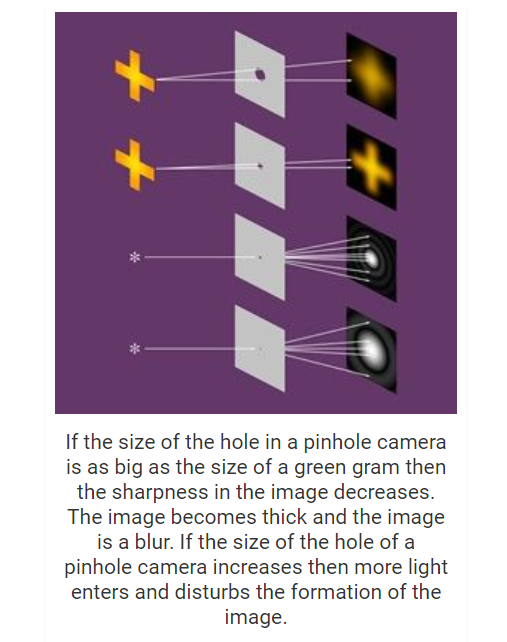17. Explain the refraction of light through a glass-slab with a neat ray diagram.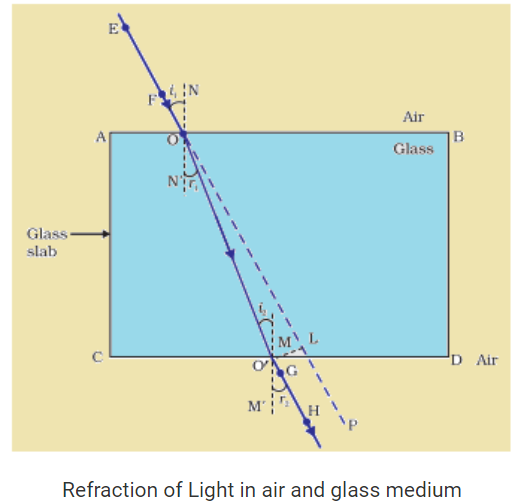Find more explanations about refraction of light in plain glass here.

18. How do you verify that the resistance of a conductor is proportional to the length of the conductor for constant cross-section area and temperature?

Answer: Electrical resistance is directly proportional to length (L) of the conductor and inversely proportional to the cross-sectional area (A). It is given by the following relation R = ρL / A, where ρ is the resistivity of the material (measured in Ω m, ohm meter).

Group-B

19. How do chemical displacement reactions differ from chemical decomposition reactions? Explain with an example for each.

Answer: In a displacement reaction, the atom or a set of atoms is displaced by another atom in a molecule. One element takes place with another element in the compound. For instance, when Iron is added to a copper sulphate solution, it displaces the copper metal.

A + B-C → A-C + B

Fe(s) + CuSO4(aq) → Cu + FeSO4

The above equation exists when A is more reactive than B.

A and B have to be either:

• Halogens where C indicates a cation.
• Different metals wherein C indicates an anion.

Meanwhile, in a chemical decomposition reaction, one reactant breaks down into two or more products. The opposite of a combination reaction – a complex molecule breaks down to make simpler ones. The general format of a decomposition reaction is provided below.

AB → A + B, where AB is the parent molecule (reactant) and A & B are the product molecules. One example for this is the reaction, NaCl→ Na + Cl.

20. Explain Hund’s rule with an example.

1. In a sublevel, each orbital is singly occupied before it is doubly occupied.

2. The electrons present in singly occupied orbitals possess identical spin.

21. Explain the formation of BF3 molecule using hybridisation.

Answer: Hybridisation is the concept of mixing two atomic orbitals with the same energy levels to give a degenerated new type of orbitals. This intermixing is based on quantum mechanics. Learn more about the hybridisation of BF3
from here.

22. Suggest a test to find the hardness of water and explain its procedure.

Answer: Hard water has high mineral content and is formed when water percolates through the deposits of chalk and limestone, which are made up of magnesium and calcium carbonates. It does not lather with soap, so it is not suitable for laundry purposes. Now, below is the procedure for a test to find the hardness of water:

i) Take 10ml of distilled water and 10ml of hard water (from hand pump) in separate test tubes.

ii) Add a couple of drops of soap solution to both.

iii) Shake the test tubes vigorously for an equal period of time and observe the foam formed.

iv) The test tube containing distilled water shows more foam. This is because the whole of soap is available to form foam.

v) The test tube containing hand-pump water may show a white curd like precipitate, indicating the presence of soluble salts of calcium or magnesium.

vi) Calcium and Magnesium ions form precipitate with soap and this indicates that water is hard water

Section-IV 5 X 1= 5

23. Draw a neat diagram of the electric motor and name the parts.

Answer: For diagram of the electric motor with its parts and functions check here.

24. Draw a diagram showing froth floatation method and label its parts.

Answer: For the labelled diagram indicating the froth floatation method and its process, check here.

Part-B

I 20 X ½ = 10

1. The boiling point of water at normal atmospheric pressure is ______

1. 0 °C
2. 100 °C
3. 110 °C
4. – 4 °C

2. Magnification(m)= _____

1. v/u
2. u/v
3. h0/hi
4. hi/h0

3. If an object is placed at ‘C’ of a concave mirror, the position of the image is______

1. at infinity
2. between F and C
3. at C
4. beyond C

4. The refractive index of glass with respect to air is 2. Then, the critical angle of glass-air interface is _______

(A) 0 °C

(B) 45 °C

(C) 30 °C

(D) 60 °C

5. Which one of the following materials cannot be used to make lens?

1. Water
2. Glass
3. Plastic
4. Clay

6. During refraction_________ will not change.

1. Wavelength
2. Frequency
3. Speed of light
4. All the above

7. A charge is moved from point A to point B. The work done to move unit during this process, is ______________

1. Potential at A
2. Potential at B
3. Current from A to B
4. Potential difference between A and B

Answer: (D) Potential difference between A and B

8. A thick wire has ______ resistance than a thin wire

1. more
2. less
3. equal
4. A and B

9. What converts mechanical energy into electrical energy?

1. Motor
2. Battery
3. Generator
4. Switch

10. The SI unit of magnetic field induction is _______

1. Tesla
2. Weber
3. Weber/m
4. Weber.m

11. C6H12O6 →C2H5OH +CO2 is _______ chemical reaction.

1. combination
2. decomposition
3. displacement
4. double decomposition

12. ______ is used for treating indigestion.

1. antibiotic
2. analgesic
3. antacid
4. antiseptic

13. Colour of Methyl orange in alkali condition is_________

1. orange
2. yellow
3. red
4. blue

14. The maximum number of electrons present in the K shell are_____

1. 2
2. 4
3. 6
4. 8

15. The value of Planck’s constant is____

1. 6.023 X 10-34 Js
2. 6.626 X 1034 Js
3. 6.626 X 10-36Js
4. None

16. Number of elements present in period 1 are_______

1. 2
2. 4
3. 6
4. 8

17. Which of the following elements is electronegative?

1. Sodium
2. Oxygen
3. Magnesium
4. Calcium

18. The bond angle in Methane _____

1. 104° 31’
2. 107° 48’
3. 180°
4. 109° 28’

19. The reducing agent in Thermite process is _______

1. Al
2. Mg
3. Fe
4. Si

20. Which of the following hydrocarbons can show isomerism?

1. C2H4
2. C2H6
3. C3H8
4. C4H10

II 5 X ½ = 2 ½

21. The geometric centre of mirror is ___________

22. At critical angle of incidence, the angle of refraction is______

23. The lens which can form real and virtual images is ______

24. The kilowatt hour is the unit of _______

25. Faraday’s Law of Induction is the consequence of _________